# Waiting room

In the waiting room are people and flies. Together they have 15 heads and 50 legs (fly has 6 legs). How many people and flies are in the waiting room?

Correct result:

x =  5
y =  9

#### Solution:

$y=9$

x+y=14; 2x+6y=64

x+y=14
2•x+6•y=64

x+y = 14
2x+6y = 64

x = 5
y = 9

Calculated by our linear equations calculator.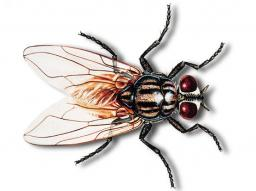We would be very happy if you find an error in the example, spelling mistakes, or inaccuracies, and please send it to us. We thank you!Tips to related online calculators
Do you have a system of equations and looking for calculator system of linear equations?

## Next similar math problems:

• The dormitoryThe dormitory accommodates 150 pupils in 42 rooms, some of which are triple and some are quadruple. Determine how many rooms are triple and how many quadruples.
• Hotel roomsIn the 45 rooms, there were 169 guests, some rooms were three-bedrooms and some five-bedrooms. How many rooms were?
• Coins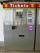In ticket maschine were together one hundred coins. They were only 20 and 50 cent coins. The sum total was 29 euros and 60 cents. How many were in ticket maschine coins and which type?
• MushroomsEva and Jane collected 114 mushrooms together. Eve found twice as much as Jane. How many mushrooms found each of them?
• Jane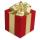Jane buy gift together for 464 Sk. For tie paid 4.5 times less than in the shirt, but 56 Sk more than for socks. How much she paid for each item in gift?
• Two numbersWe have two numbers. Their sum is 140. One-fifth of the first number is equal to half the second number. Determine those unknown numbers.
• Three brothersThe three brothers have a total of 42 years. Jan is five years younger than Peter and Peter is 2 years younger than Michael. How many years has each of them?
• EquationsSolve following system of equations: 6(x+7)+4(y-5)=12 2(x+y)-3(-2x+4y)=-44
• Linear systemSolve this linear system (two linear equations with two unknowns): x+y =36 19x+22y=720
• Equations - simpleSolve system of linear equations: x-2y=6 3x+2y=4
• TreesAlong the road were planted 250 trees of two types. Cherry for 60 CZK apiece and apple 50 CZK apiece. The entire plantation cost 12,800 CZK. How many was cherries and apples?
• TheatroTheatrical performance was attended by 480 spectators. Women were in the audience 40 more than men and children 60 less than half of adult spectators. How many men, women and children attended a theater performance?
• Magic numberThe number 135 split to two addends so that one addend was 30 greater than 2/5 the addend.
• Tickets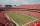On the football tournament ticket cost 45 Kc for standing and 120kč for sitting. Sitting spectators was 1/3 more than standing. The organizers collected a total 12 300 Kc. How many seated and standing seats (spectators)?
• Brunette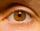Of the girls who attend primary school are 30% blondes and 70% brunette. 81% blondes and 19% brunette has blue eyes. How many girls attending school altogether if 470 girls has blue eyes?Number 118 divide into two addends, so first addend is 69 greater than 75% of the second addend.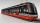In the three-part tram went 206 passengers in front of others, 226 after others and in the middle half of all. How many passengers went in tram total?